EN | ES | DE | NL | RU

## Gas Laws with Examples

Gas Laws with Examples

1. Boyle's Law:(Pressure-volume relation)

Gases have property of expansion and compressibility. Types of gas does not affect ratio of expansion or compressibility. All gases has same expansion constant. We can define Boyle's Law;

"Under constant temperature and number of particles, pressure and volume of gases are inversely proportional to each other."

V is inversely proportional to P or

P.V=constant

Moreover;

P1.V1=P2.V2=P3.V3=.. (for same gas under constant temperature and number of particles.)

Following picture summarizes boyle's law;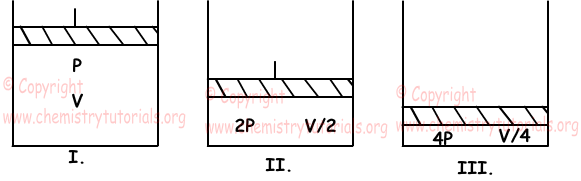In first container we have P.V

In second container we have 2P.V/2=P.V

In third container  we have 4P.V/4=P.V

As you can see; as we decrease the volume of container, pressure of gas increases with same amount and multiplication of P and V is always constant.

Example: Gas having 150 cm3 volume has pressure 120 cmHg. If we increase volume of container to 300 cm3, find final pressure of the gas.

Since P1.V1 is constant from boyle's law;

P1.V1=P2.V2

120.150=P2.300

P2=60 cm Hg

As you can see from the example, as we increase volume of gas, pressure decreases with same amount.

2. Charles' Law:(Volume-temperature relation)

Under constant number of particles and pressure, volume of gas is directly proportional to absolute temperature. This statement is called "Charles' law".

V/T=constant (number of particles "n" and pressure constant "P")

Moreover, in one situation, ratio of V/T is equal to V1/T1 in another situation for same gas under constant n and P. Following graph shows relation between volume and temperature of gases under constant pressure and number of particles.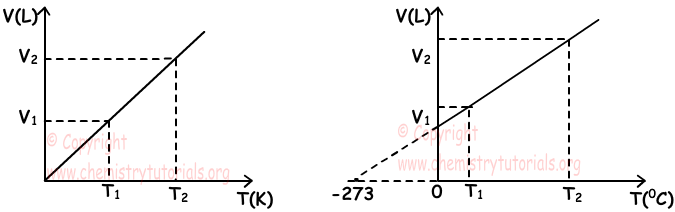Examine graphs given above. We must take temperature in K unit always you can see the changes in the graphs when we take 0C as unit and K as unit.

Example: Gas at 127 0C has volume 240 ml. If we increase temperature of gas from 127 0C to 227 0C, find final volume of the gas.

Solution:

We first convert unit of temperature.

T1=127+273=400 K

T2=227+273=500 K

V1=240 ml

V2=?

We use Charles' law to solve this problem.

V1/T1=V2/T2

240/400=V2/500

V2=300 ml

Be careful, if you do not change unit of temperature, you can not find real value of volume.

3. Gay Lussac's / Amonton's Law:(Pressure-temperature relation)

When we increase temperature of gas, placed in a container having constant volume, speed of gas molecules increase. Increasing in the speed of molecules increase collision number to surfaces this is pressure. In other words, increasing temperature of the gas under constant volume and number of particles, increase the pressure of gas. Graphs given below show pressure temperature relation of gas under constant n and V.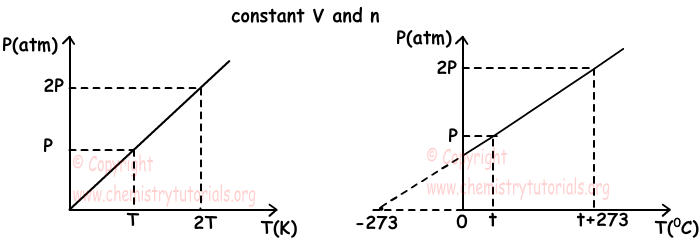To sum up we can write following equation;

P1/T1=constant

Thus; P1/T1=P2/T2

Example: If we want to decrease pressure of gas, placed in a container having constant volume, from 4P to P how much we should change the temperature of it. Its current temperature is 127 0C.

P1=4P

P2=P

T1=127 0C=127+273=400 K

P1/T1=P2/T2

4P/400=P/T2

T2=100 K=t+273

t=-173 0C

4. Avogadro's Law: (Volume-Number of particles relation)

Gases, having equal pressure and temperature, have equal number of particles in equal amount of volumes. In other words, volume and number of particles of gases are directly proportional to each other. We said in previous topics that 1mol gas is 22,4 liter under standard pressure and temperature and 1mol gas contains 6,02x1023 molecules/atoms. We can summarize this relation with following equation;

V/n=constant or;

V1/n1=V2/n2 (P and T are constant)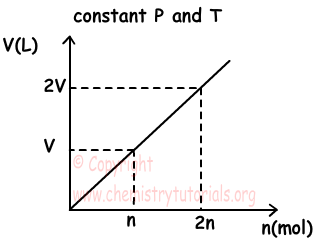Example: If 5g O2 gas has volume 200 cm3, find volume of 20 cm3 O2 under same conditions. (O=16)

Solution:

O2=2x16=32

we should find moles of O2 in two situation.

n1=5/32 moles and n2=20/32 moles

V1/n1=V2/n2

200/5/32=V2/20/32

V2=800 cm3

5. Dalton's Law:(Pressure-number of particles relation)

Increasing number of particles in a closed container, pressure of gas increases. In other words, pressure of gases is directly proportional to moles of it under constant volume and temperature.

P/n=constant or;

P1/n1=P2/n2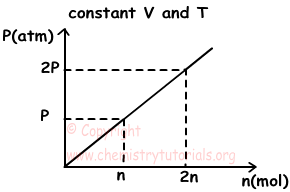Example: If pressure of SO2 decreases from 6P to 3P, find change in the moles of it under constant volume and temperature.

Solution:

P1=6P, P2=3P

n1=n

n2=?

Using Dalton's Law;

P1/n1=P2/n2

6P/n=3P/n2

n2=n/2

change in the moles of SO2 is n-n/2=n/2

The Original Author:

© Copyright www.ChemistryTutorials.org, Reproduction in electronic and written form is expressly forbidden without written permission of www.ChemistryTutorials.org. Privacy Policy